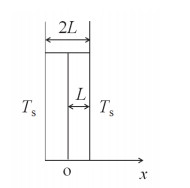## Science in Hot Pot: Determining the Heat-Up Duration Using Model of Unsteady Heat Transfer

, ,,Abstract

Hot pot is a famous traditional Chinese cuisine. During hot pot, people usually judge whether or not the meat is well done by observation. This way for making judgement lacks scientific support and may possibly cause safety risks. In this paper, we set up two models for meat slice and ball, respectively, and treated the heat transfer from soup to the very center of meat based on these unsteady state heat transfer models. The temperature of very center of meat is plotted against time, and the time required for heating the very center of meat to ca. 100℃ was determined. It was found that the time required for the center temperature to attain 100℃ is ca. 15 s for meat slice of 1.8 mm thick, and more than 8 min for meat ball of 2.6 cm in diameter. The discussion and conclusion are helpful for food safety.

Keywords： Hot pot ; Unsteady heat transfer ; Plane model ; Spherical model

LI Xiaoyu, LIU Ke, ZHANG Shuyong. Science in Hot Pot: Determining the Heat-Up Duration Using Model of Unsteady Heat Transfer. University Chemistry[J], 2019, 34(1): 72-76 doi:10.3866/PKU.DXHX201804037

## 1 引言

### 图1$\frac{\text{1}}{\alpha }\frac{\partial T}{\partial t}\text{=}{{\nabla }^{\text{2}}}T+\frac{q}{k}$

$\frac{\partial T}{\partial t}\text{=}\alpha \frac{{{\partial }^{\text{2}}}T}{\partial {{x}^{\text{2}}}}$

$t=\text{0}, T ={{T }_{\text{0}}}$

$x~=\pm L;\ \ T~={{T}_{\text{s}}}$

$x=\text{0;} \frac{\partial t}{\partial x}=\text{0}$

${{T}^{\text{*}}}=\frac{T-{{T}_{\text{s}}}}{{{T}_{0}}-{{T}_{\text{s}}}}$

${{L}^{*}}\text{=}\frac{x}{L}$

$\frac{\partial {{T}^{*}}}{\partial {{F}_{\text{o}}}}=\frac{{{\partial }^{2}}{{T}^{\text{*}}}}{\partial {{L}^{*}}^{2}}$

${{F}_{\text{o}}}=0;\ \ {{T}^{\text{*}}}=1$

${{L}^{\text{*}}}=\text{1;} \;\;{{T}^{*}}=\text{0}$

${{L}^{\text{*}}}=\text{0;}\ \ \;\;\frac{\partial {{T}^{\text{*}}}}{\partial {{L}^{\text{*}}}}=\text{0}$

\begin{align} &{{T}^{*}}\text{=}\frac{\text{4}}{\text{ }\!\!\pi\!\!\text{ }}\{\text{exp}[\text{-}{{\left( \pi /\text{2} \right)}^{\text{2}}}{{F}_{\text{o}}}]\text{cos}\left( \frac{\pi }{2}{{L}^{*}} \right)-\frac{\text{1}}{\text{3}}\text{exp}[\text{-}{{\left( \text{3}\pi /\text{2} \right)}^{\text{2}}}{{F}_{\text{o}}}]\text{cos}\left( \frac{\text{3}\pi }{\text{2}}{{L}^{*}} \right)+ \\ & \frac{\text{1}}{\text{5}}\text{exp}[\text{-}{{\left( \text{5}\pi /\text{2} \right)}^{\text{2}}}{{F}_{\text{o}}}]\text{cos}\left( \frac{\text{5}\pi }{\text{2}}{{L}^{*}} \right)-...\} \\ \end{align}

$\alpha \text{=}\frac{\lambda }{C\times \rho }\text{=}\frac{\text{0}\text{.50}}{\text{3204}\times \text{1170}}\text{=1}\text{.30}\times \text{1}{{\text{0}}^{-\text{7}}}\text{ }{{\text{m}}^{\text{2}}}\cdot {{\text{s}}^{-\text{1}}}$

${{F}_{\text{o}}}\text{ = }\frac{\alpha }{{{L}^{\text{2}}}}t\text{ = }\frac{\text{1}\text{.3}\times \text{1}{{\text{0}}^{-\text{7}}}}{{{\left( \text{0}\text{.9}\times \text{1}{{\text{0}}^{-\text{3}}} \right)}^{\text{2}}}}t\text{ = 0}\text{.1605}t$

### 图2### 2.2 球状食物的传热

$\frac{\partial T}{\partial t}\text{=}\frac{\alpha }{{{r}^{\text{2}}}}\frac{\partial }{\partial r}\left( {{r}^{\text{2}}}\frac{\partial T}{\partial r} \right)$

$t=\text{ 0 ;}\ \ T={{T}_{\text{0}}}$

$r=\pm {{R}_{\text{0}}}; \;T={{T}_{\text{s}}}$

$r=\text{0;}\ \text{ }\frac{\partial T}{\partial r}=\text{0}$

${{T}^{*}}=\frac{T-{{T}_{\text{s}}}}{{{T}_{\text{0}}}-{{T}_{\text{s}}}}$

${{X}^{*}}=\frac{r}{{{R}_{\text{0}}}}$

${{n}^{\text{*}}}=\frac{\alpha }{{{R}_{\text{0}}}^{\text{2}}}t$

$\frac{\partial {{T}^{*}}}{\partial {{n}^{*}}}=\frac{\text{1}}{{{X}^{\text{*2}}}}\frac{\partial }{\partial {{X}^{*}}}\left( {{X}^{\text{*2}}}\frac{\partial {{T}^{*}}}{\partial {{X}^{*}}} \right)$

${{n}^{*}}=\text{0;}\ \ {{T}^{*}}=\text{1}$

${{X}^{*}}=\text{1; }{{T}^{*}}=\text{0}$

${{X}^{*}}=\text{0;}\ \frac{\partial {{T}^{*}}}{\partial {{X}^{*}}}=\text{0}$

${{T}^{*}}=\frac{\text{2}}{\pi {{X}^{*}}}\{\text{exp}[-{{\pi }^{\text{2}}}{{n}^{\text{*}}}]\text{sin}\left( \pi {{X}^{*}} \right)-\frac{\text{1}}{\text{2}}\text{exp }\!\![\!\!\text{ }-\text{4}{{\pi }^{2}}n\text{ }\!\!]\!\!\text{ sin}\left( \text{4}\pi {{X}^{*}} \right)+\frac{\text{1}}{\text{3}}\text{exp}[-\text{9}{{\pi }^{\text{2}}}{{n}^{*}}]\text{sin}\left( 3\pi {{X}^{*}} \right) +...\}$

${{n}^{*}}=\frac{\alpha }{R_{\text{0}}^{\text{2}}}t=\frac{\text{1}\text{.3}\times \text{1}{{\text{0}}^{-\text{7}}}}{{{\left( \text{1}\text{.3}\times \text{1}{{\text{0}}^{-\text{2}}} \right)}^{\text{2}}}}t=\text{7}\text{.692}\times \text{1}{{\text{0}}^{-\text{4}}}t$

### 图3## 参考文献 原文顺序 文献年度倒序 文中引用次数倒序 被引期刊影响因子

[2018-03-22]. http://health.people.com.cn/n1/2017/1013/c14739-29585266.html.

/

 〈〉